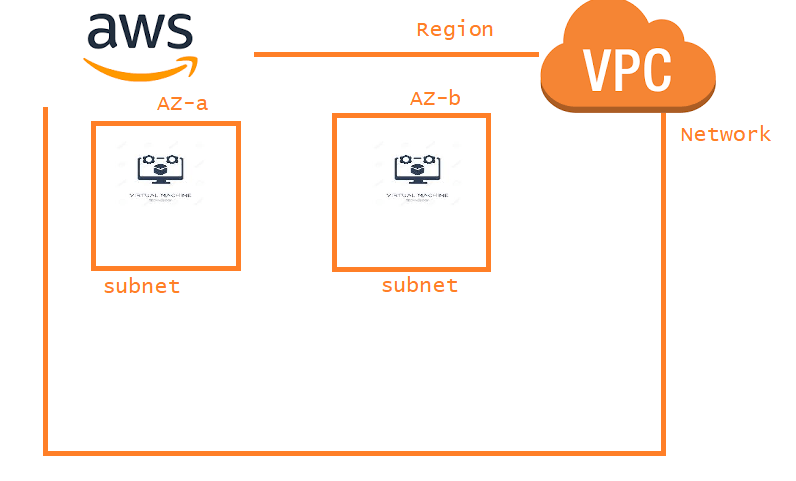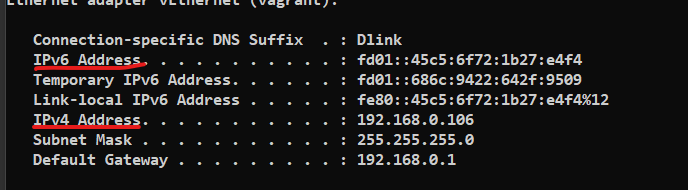### How about networking in AWS

• In AWS the networks which we will be creating are virtual.
• From 5000 feet overview how would aws network which we create look like
• In AWS we create network at the region level which we call as vpc (Virtual Private Cloud), But we know that infrastructure in AWS is in Availability zones (AZ’s).
• So we create subnets at AZ level. This is where we add resources like virtual machines, databases etc to our network### Basics

• Networking principles:
1. A device on a network can send messages to any other device on the same network
• It is a numeric label assigned to each device connected to a computer network.
• IP address helps in host and network identification.
• IP address is combination of network id and host id
• Two devices on the same network will have same network id and different host ids
• IP address has two versions
• IPv4:
• defines IP address as 32 bit number
• Eg: 192.168.10.11
• Can serve for 2^32 addresses (We cannot use all the ip addresss as there are some reservations)
• IPv6:
• defines IP address as 128 bit number
• Eg: 2001:da8:0:1234:0:678:9:1
• Can serve for 2^128 addresses• An IPv4 address is represented as `x.x.x.x` each x represent 8 bits or a byte
• each position can have a range of 0-(2^8-1) i.e 0-255 so ipv4 range would 0.0.0.0 to 255.255.255.255
• In the above image along with ipaddress we also have subnet mask and Default gateway, so are they required
• IP address is combination of network id + host id, to determine what is network id and what is host id we need subnet mask.
``````
1   2  3  4

What are positions where we see 255 => 1,2,3
network id => 192.168.0.0
host id => 106
size of network => what is size of host id => 1 byte => 8 bits
=> 2^n -2 => 2^8-2 => 254

network id => 10.12.0.0
host id => 0.5

size of network => 2 bytes => 16 bits => 2^16-2 => 65536 - 2 => 65534
``````
• Now if you are asked to create a network with capacity of 500 devices, how will i decide on subnet mask. To help in creating networks a classful addressing defintion can be used Refer Here
• Class A => 255.0.0.0 => 2^24 ~= 16,777,216
• Class B => 255.255.0.0 => 2^16 ~= 65536
• Class C => 255.255.255.0 => 2^8 = 256
• For 500 devices if i follow classful network i have to use Class B. Do you think it is a good idea to create class B (65000) for 500 devices
• To resolve this CIDR (Classless interdomain routing) was introduced

#### How CIDR tries to solve the problem

• In classful addressing subnet masks are consider as decimal number, what cidr tries is look at subnet mask as a binary number,
``````ip address: 192.168.0.106
255.255.255.0

How many consecutive 1's you have from starting?

24

What is left for hosts => 32-24 => 8 or count zeros =>8

size of network 2^8-2 = 254
In cidr this ip address is written as 192.168.0.106/24

255.255.254.0

How many consecutive 1's you have from starting?
23
What is left for hosts => 32-23=> 9 or count zeros =>9
size of network => 2^9-2 = 510
cidr notation => 192.168.0.106/23

``````
• possible subnet values for 1 byte
``````11111111 => 255
11111110 => 254
11111100 => 252
11111000 => 248
11110000 => 240
11100000 => 224
11000000 => 192
10000000 => 128

``````

This site uses Akismet to reduce spam. Learn how your comment data is processed.